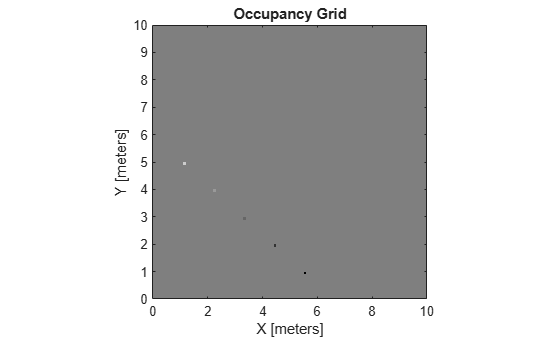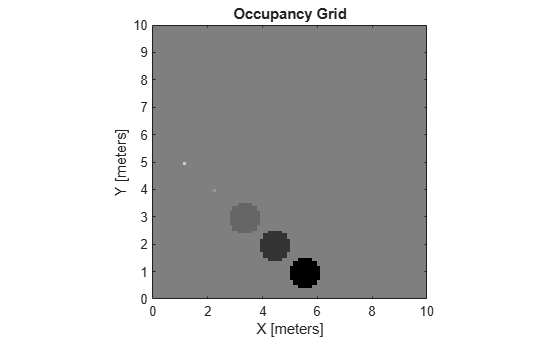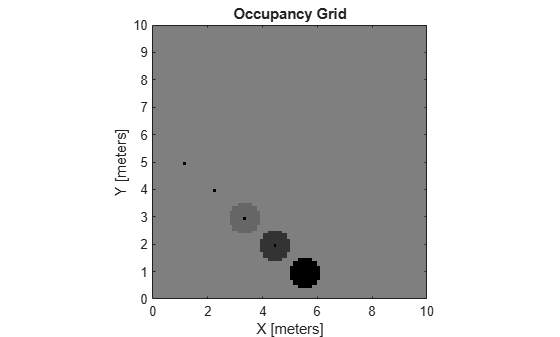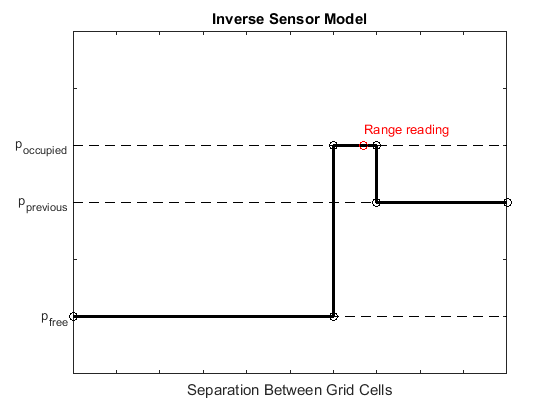# updateOccupancy

Integrate probability observations at locations

## Syntax

``updateOccupancy(map,occMatrix)``
``updateOccupancy(map,locations,obs)``
``updateOccupancy(map,xy,obs,'world')``
``updateOccupancy(map,xy,obs,'local')``
``updateOccupancy(map,ij,obs,'grid')``
``updateOccupancy(map,bottomLeft,obsMatrix)``
``updateOccupancy(map,bottomLeft,obsMatrix,'world')``
``updateOccupancy(map,bottomLeft,obsMatrix,'local')``
``updateOccupancy(map,topLeft,obsMatrix,'grid')``

## Description

````updateOccupancy(map,occMatrix)` probabilistically integrates a matrix of occupancy values, `occMatrix`, with the current occupancy matrix of the `occupancyMap` object `map`. The size of the matrix must be equal to the `GridSize` property of map.```

example

````updateOccupancy(map,locations,obs)` probabilistically integrates observation values, `obs`, into the occupancy map cells corresponding to the n-by-2 matrix of world coordinates `locations`. Observation values are determined based on the Inverse Sensor Model.```
````updateOccupancy(map,xy,obs,'world')` probabilistically integrates observation values, `obs`, into the cells corresponding to the n-by-2 matrix of world coordinates `xy`.```
````updateOccupancy(map,xy,obs,'local')` probabilistically integrates observation values, `obs`, into the cells corresponding to the n-by-2 matrix of local coordinates `xy`.```
````updateOccupancy(map,ij,obs,'grid')` probabilistically integrates observation values, `obs`, into the cells corresponding to the n-by-2 matrix of grid indices `ij`.```
````updateOccupancy(map,bottomLeft,obsMatrix)` probabilistically integrates an m-by-n matrix of observation values, `obsMatrix`, into a subregion in the map. Specify the bottom-left corner of the subregion as a world position, `bottomLeft`. The subregion extends m rows up and n columns to the right from the specified position.```
````updateOccupancy(map,bottomLeft,obsMatrix,'world')` probabilistically integrates an m-by-n matrix of observation values, `obsMatrix`, into a subregion in the map. Specify the bottom-left corner of the subregion as a world position, `bottomLeft`. The subregion extends m rows up and n columns to the right from the specified position.```
````updateOccupancy(map,bottomLeft,obsMatrix,'local')` probabilistically integrates an m-by-n matrix of observation values, `obsMatrix`, into a subregion in the map. Specify the bottom-left corner of the subregion as a local position, `bottomLeft`. The subregion extends m rows up and n columns to the right from the specified position.```
````updateOccupancy(map,topLeft,obsMatrix,'grid')` probabilistically integrates an m-by-n matrix of observation values, `obsMatrix`, into a subregion in the map. Specify the top-left corner of the subregion as a grid index, `topLeft`. The subregion extends m rows down and n columns to the right from the specified index.```

## Examples

collapse all

Create an empty map of 10-by-10 meters in size.

`map = occupancyMap(10,10,10);`

Update the occupancy of specific world locations with new probability values and display the map.

```x = [1.2; 2.3; 3.4; 4.5; 5.6]; y = [5.0; 4.0; 3.0; 2.0; 1.0]; pvalues = [0.2; 0.4; 0.6; 0.8; 1]; updateOccupancy(map,[x y],pvalues) figure show(map)```Inflate the occupied areas by a radius of 0.5 m. The larger occupancy values overwrite the smaller values.

```inflate(map,0.5) figure show(map)```Get the grid locations from the world locations.

`ij = world2grid(map,[x y]);`

Set occupancy values for the grid locations.

```setOccupancy(map,ij,ones(5,1),'grid') figure show(map)```## Input Arguments

collapse all

Map representation, specified as an `occupancyMap` object. This object represents the environment of the vehicle. The object contains a matrix grid with each value representing the probability of the occupancy of that cell. Values close to `1` represent a high probability that the cell contains an obstacle. Values close to `0` represent a high probability that the cell is not occupied and contains no obstacles.

Matrix of occupancy values, specified as a matrix. The size of the matrix must be equal to the `GridSize` property of map.

The occupancy values can be of any numeric type, with values between 0 and 1. If the matrix is logical, the default occupancy values of 0.7 (`true`) and 0.4 (`false`) are used.

Example: `updateOccupancy(map,ones(map.GridSize)*0.6)`

Data Types: `single` | `double` | `logical`

Cell locations in world coordinates, specified as an n-by-2 matrix with rows of the form [x y], where n is the number of world coordinates. The function ignores locations outside of the map boundaries.

Example: ```updateOccupancy(map,[1 1; 3 3; 5 5],false)```

Data Types: `single` | `double`

Location of the bottom-left corner of the observation matrix, specified as a two-element vector of the form ```[xCoord yCoord]```. The location is in world or local coordinates, based on the syntax.

Example: ```updateOccupancy(map,[2 2],[0.2 0.4; 0.6 0.8],'world')```

Data Types: `single` | `double`

Location of the top-left corner of the grid, specified as a two-element vector of form `[iCoord jCoord]`.

Example: ```updateOccupancy(map,[2 2],[0.2 0.4; 0.6 0.8],'grid')```

Data Types: `single` | `double`

World or local coordinates, specified as an n-by-2 matrix with rows of the form ```[x y]```, where n is the number of coordinates.

Example: ```updateOccupancy(map,[2 2; 4 4; 6 6],[0.2; 0.4; 0.6],'world')```

Data Types: `single` | `double`

Grid positions, specified as an n-by-2 matrix with rows of the form ```[i j]``` in ```[rows cols]``` format, where n is the number of grid positions.

Example: ```updateOccupancy(map,[2 2; 4 4; 6 6],[0.2; 0.4; 0.6],'grid')```

Data Types: `single` | `double`

Probability observation values, specified as a numeric or logical scalar or a numeric or logical n-element column vector the same size as either `locations`, `xy`, or `ij`.

`obs` values can be any value from 0 to 1, but if `obs` is a logical vector, the default observation values of 0.7 (`true`) and 0.4 (`false`) are used. If `obs` is a numeric or a logical scalar, the value is applied to all coordinates in `locations`, `xy`, or `ij`. These values correlate to the Inverse Sensor Model for ray casting.

Example: ```updateOccupancy(map,[2 2; 4 4; 6 6],[0.2; 0.4; 0.6],'local')```

Data Types: `single` | `double` | `logical`

Matrix of probability observation values, specified as an m-by-n numeric or logical matrix.

The observation values can be of any numeric type with value between 0 and 1. If the matrix is logical, the default observation values of 0.7 (`true`) and 0.4 (`false`) are used.

Example: ```updateOccupancy(map,[2 2],[0.2 0.4; 0.6 0.8])```

Data Types: `single` | `double` | `logical`

collapse all

### Inverse Sensor Model

The inverse sensor model determines how values are set along a ray from a range sensor reading to the obstacles in the map. `NaN` range values are ignored. Range values greater than `maxrange` are not updated.Grid locations that contain range readings are updated with the occupied probability. Locations before the reading are updated with the free probability. All locations after the reading are not updated.